# lassoblm

Bayesian linear regression model with lasso regularization

## Description

The Bayesian linear regression model object `lassoblm` specifies the joint prior distribution of the regression coefficients and the disturbance variance (β, σ2) for implementing Bayesian lasso regression . For j = 1,…,`NumPredictors`, the conditional prior distribution of βj|σ2 is the Laplace (double exponential) distribution with a mean of 0 and scale σ2/λ, where λ is the lasso regularization, or shrinkage, parameter. The prior distribution of σ2 is inverse gamma with shape A and scale B.

The data likelihood is $\prod _{t=1}^{T}\varphi \left({y}_{t};{x}_{t}\beta ,{\sigma }^{2}\right),$ where ϕ(yt;xtβ,σ2) is the Gaussian probability density evaluated at yt with mean xtβ and variance σ2. The resulting posterior distribution is not analytically tractable. For details on the posterior distribution, see Analytically Tractable Posteriors.

In general, when you create a Bayesian linear regression model object, it specifies the joint prior distribution and characteristics of the linear regression model only. That is, the model object is a template intended for further use. Specifically, to incorporate data into the model for posterior distribution analysis and feature selection, pass the model object and data to the appropriate object function.

## Creation

### Syntax

``PriorMdl = lassoblm(NumPredictors)``
``PriorMdl = lassoblm(NumPredictors,Name,Value)``

### Description

example

``` `PriorMdl = lassoblm(NumPredictors)` creates a Bayesian linear regression model object (`PriorMdl`) composed of `NumPredictors` predictors and an intercept, and sets the `NumPredictors` property. The joint prior distribution of (β, σ2) is appropriate for implementing Bayesian lasso regression . `PriorMdl` is a template that defines the prior distributions and specifies the values of the lasso regularization parameter λ and the dimensionality of β.```

example

``` `PriorMdl = lassoblm(NumPredictors,Name,Value)` sets properties (except `NumPredictors`) using name-value pair arguments. Enclose each property name in quotes. For example, `lassoblm(3,'Lambda',0.5)` specifies a shrinkage of `0.5` for the three coefficients (not the intercept).```

## Properties

expand all

You can set writable property values when you create the model object by using name-value pair argument syntax, or after you create the model object by using dot notation. For example, to set the shrinkage for all coefficients, except the intercept, to `0.5`, enter

`PriorMdl.Lambda = 0.5;`

Number of predictor variables in the Bayesian multiple linear regression model, specified as a nonnegative integer.

`NumPredictors` must be the same as the number of columns in your predictor data, which you specify during model estimation or simulation.

When specifying `NumPredictors`, exclude any intercept term for the value.

After creating a model, if you change the of value `NumPredictors` using dot notation, then these parameters revert to the default values:

• Variable names (`VarNames`)

• Shrinkage parameter (`Lambda`)

Data Types: `double`

Flag for including a regression model intercept, specified as a value in this table.

ValueDescription
`false`Exclude an intercept from the regression model. Therefore, β is a `p`-dimensional vector, where `p` is the value of `NumPredictors`.
`true`Include an intercept in the regression model. Therefore, β is a (`p` + 1)-dimensional vector. This specification causes a T-by-1 vector of ones to be prepended to the predictor data during estimation and simulation.

If you include a column of ones in the predictor data for an intercept term, then set `Intercept` to `false`.

Example: `'Intercept',false`

Data Types: `logical`

Predictor variable names for displays, specified as a string vector or cell vector of character vectors. `VarNames` must contain `NumPredictors` elements. `VarNames(j)` is the name of the variable in column `j` of the predictor data set, which you specify during estimation, simulation, or forecasting.

The default is `{'Beta(1)','Beta(2),...,Beta(p)}`, where `p` is the value of `NumPredictors`.

Example: `'VarNames',["UnemploymentRate"; "CPI"]`

Data Types: `string` | `cell` | `char`

Lasso regularization parameter for all regression coefficients, specified as a positive numeric scalar or (`Intercept` + `NumPredictors`)-by-1 positive numeric vector. Larger values of `Lambda` cause corresponding coefficients to shrink closer to zero.

Suppose `X` is a `T`-by-`NumPredictors` matrix of predictor data, which you specify during estimation, simulation, or forecasting.

• If `Lambda` is a vector and `Intercept` is `true`, `Lambda(1)` is the shrinkage for the intercept, `Lambda(2)` is the shrinkage for the coefficient of the first predictor `X(:,1)`, `Lambda(3)` is the shrinkage for the coefficient of the second predictor `X(:,2)`,…, and ```Lambda(NumPredictors + 1)``` is the shrinkage for the coefficient of the last predictor `X(:,NumPredictors)`.

• If `Lambda` is a vector and `Intercept` is `false`, `Lambda(1)` is the shrinkage for the coefficient of the first predictor `X(:,1)`,…, and `Lambda(NumPredictors)` is the shrinkage for the coefficient of the last predictor `X(:,NumPredictors)`.

• If you supply the scalar `s` for `Lambda`, then all coefficients of the predictors in `X` have a shrinkage of `s`.

• If `Intercept` is `true`, the intercept has a shrinkage of `0.01`, and `lassoblm` stores ```[0.01; s*ones(NumPredictors,1)]``` in `Lambda`.

• Otherwise, `lassoblm` stores `s*ones(NumPredictors,1)` in `Lambda`.

Example: `'Lambda',6`

Data Types: `double`

Shape hyperparameter of the inverse gamma prior on σ2, specified as a numeric scalar.

`A` must be at least ```–(Intercept + NumPredictors)/2```.

With `B` held fixed, the inverse gamma distribution becomes taller and more concentrated as `A` increases. This characteristic weighs the prior model of σ2 more heavily than the likelihood during posterior estimation.

For the functional form of the inverse gamma distribution, see Analytically Tractable Posteriors.

Example: `'A',0.1`

Data Types: `double`

Scale parameter of inverse gamma prior on σ2, specified as a positive scalar or `Inf`.

With `A` held fixed, the inverse gamma distribution becomes taller and more concentrated as `B` increases. This characteristic weighs the prior model of σ2 more heavily than the likelihood during posterior estimation.

Example: `'B',5`

Data Types: `double`

## Object Functions

 `estimate` Perform predictor variable selection for Bayesian linear regression models `simulate` Simulate regression coefficients and disturbance variance of Bayesian linear regression model `forecast` Forecast responses of Bayesian linear regression model `plot` Visualize prior and posterior densities of Bayesian linear regression model parameters `summarize` Distribution summary statistics of Bayesian linear regression model for predictor variable selection

## Examples

collapse all

Consider the multiple linear regression model that predicts the US real gross national product (`GNPR`) using a linear combination of industrial production index (`IPI`), total employment (`E`), and real wages (`WR`).

`${\text{GNPR}}_{t}={\beta }_{0}+{\beta }_{1}{\text{IPI}}_{t}+{\beta }_{2}{\text{E}}_{t}+{\beta }_{3}{\text{WR}}_{t}+{\epsilon }_{t}.$`

For all $t$, ${\epsilon }_{t}$ is a series of independent Gaussian disturbances with a mean of 0 and variance ${\sigma }^{2}$.

Assume these prior distributions:

• For j = 0,...,3, ${\beta }_{j}|{\sigma }^{2}$ has a Laplace distribution with a mean of 0 and a scale of ${\sigma }^{2}/\lambda$, where $\lambda$ is the shrinkage parameter. The coefficients are conditionally independent.

• ${\sigma }^{2}\sim IG\left(A,B\right)$. $A$ and $B$ are the shape and scale, respectively, of an inverse gamma distribution.

Create a prior model for Bayesian linear regression. Specify the number of predictors `p`.

```p = 3; Mdl = lassoblm(p);```

`PriorMdl` is a `lassoblm` Bayesian linear regression model object representing the prior distribution of the regression coefficients and disturbance variance. At the command window, `lassoblm` displays a summary of the prior distributions.

Alternatively, you can create a prior model for Bayesian lasso regression by passing the number of predictors to `bayeslm` and setting the `ModelType` name-value pair argument to `'lasso'`.

`MdlBayesLM = bayeslm(p,'ModelType','lasso')`
```MdlBayesLM = lassoblm with properties: NumPredictors: 3 Intercept: 1 VarNames: {4x1 cell} Lambda: [4x1 double] A: 3 B: 1 | Mean Std CI95 Positive Distribution --------------------------------------------------------------------------- Intercept | 0 100 [-200.000, 200.000] 0.500 Scale mixture Beta(1) | 0 1 [-2.000, 2.000] 0.500 Scale mixture Beta(2) | 0 1 [-2.000, 2.000] 0.500 Scale mixture Beta(3) | 0 1 [-2.000, 2.000] 0.500 Scale mixture Sigma2 | 0.5000 0.5000 [ 0.138, 1.616] 1.000 IG(3.00, 1) ```

`Mdl` and `MdlBayesLM` are equivalent model objects.

You can set writable property values of created models using dot notation. Set the regression coefficient names to the corresponding variable names.

`Mdl.VarNames = ["IPI" "E" "WR"]`
```Mdl = lassoblm with properties: NumPredictors: 3 Intercept: 1 VarNames: {4x1 cell} Lambda: [4x1 double] A: 3 B: 1 | Mean Std CI95 Positive Distribution --------------------------------------------------------------------------- Intercept | 0 100 [-200.000, 200.000] 0.500 Scale mixture IPI | 0 1 [-2.000, 2.000] 0.500 Scale mixture E | 0 1 [-2.000, 2.000] 0.500 Scale mixture WR | 0 1 [-2.000, 2.000] 0.500 Scale mixture Sigma2 | 0.5000 0.5000 [ 0.138, 1.616] 1.000 IG(3.00, 1) ```

MATLAB® associates the variable names to the regression coefficients in displays.

Consider the linear regression model in Create Prior Model for Bayesian Lasso Regression.

Create a prior model for performing Bayesian lasso regression. Specify the number of predictors `p` and the names of the regression coefficients.

```p = 3; PriorMdl = bayeslm(p,'ModelType','lasso','VarNames',["IPI" "E" "WR"]); shrinkage = PriorMdl.Lambda```
```shrinkage = 4×1 0.0100 1.0000 1.0000 1.0000 ```

`PriorMdl` stores the shrinkage values for all predictors in its `Lambda` property. `shrinkage(1)` is the shrinkage for the intercept, and the elements of `shrinkage(2:end)` correspond to the coefficients of the predictors in `Mdl.VarNames`. The default shrinkage for the intercept is `0.01`, and the default is `1` for all other coefficients.

Load the Nelson-Plosser data set. Create variables for the response and predictor series. Because lasso is sensitive to variable scales, standardize all variables.

```load Data_NelsonPlosser X = DataTable{:,PriorMdl.VarNames(2:end)}; y = DataTable{:,'GNPR'}; X = (X - mean(X,'omitnan'))./std(X,'omitnan'); y = (y - mean(y,'omitnan'))/std(y,'omitnan');```

Although this example standardizes variables, you can specify different shrinkage values for each coefficient by setting the `Lambda` property of `PriorMdl` to a numeric vector of shrinkage values.

Implement Bayesian lasso regression by estimating the marginal posterior distributions of $\beta$ and ${\sigma }^{2}$. Because Bayesian lasso regression uses Markov chain Monte Carlo (MCMC) for estimation, set a random number seed to reproduce the results.

```rng(1); PosteriorMdl = estimate(PriorMdl,X,y);```
```Method: lasso MCMC sampling with 10000 draws Number of observations: 62 Number of predictors: 4 | Mean Std CI95 Positive Distribution ----------------------------------------------------------------------- Intercept | -0.4490 0.0527 [-0.548, -0.344] 0.000 Empirical IPI | 0.6679 0.1063 [ 0.456, 0.878] 1.000 Empirical E | 0.1114 0.1223 [-0.110, 0.365] 0.827 Empirical WR | 0.2215 0.1367 [-0.024, 0.494] 0.956 Empirical Sigma2 | 0.0343 0.0062 [ 0.024, 0.048] 1.000 Empirical ```

`PosteriorMdl` is an `empiricalblm` model object that stores draws from the posterior distributions of $\beta$ and ${\sigma }^{2}$ given the data. `estimate` displays a summary of the marginal posterior distributions at the command line. Rows of the summary correspond to regression coefficients and the disturbance variance, and columns correspond to characteristics of the posterior distribution. The characteristics include:

• `CI95`, which contains the 95% Bayesian equitailed credible intervals for the parameters. For example, the posterior probability that the regression coefficient of `E` (standardized) is in [-0.110, 0.365] is 0.95.

• `Positive`, which contains the posterior probability that the parameter is greater than 0. For example, the probability that the intercept is greater than 0 is 0.

By default, `estimate` draws and discards a burn-in sample of size 5000. However, a good practice is to inspect a trace plot of the draws for adequate mixing and lack of transience. Plot a trace plot of the draws for each parameter. You can access the draws that compose the distribution (the properties `BetaDraws` and `Sigma2Draws`) using dot notation.

```figure; for j = 1:(p + 1) subplot(2,2,j); plot(PosteriorMdl.BetaDraws(j,:)); title(sprintf('%s',PosteriorMdl.VarNames{j})); end```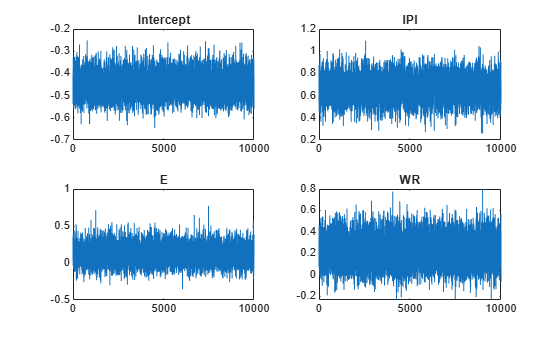```figure; plot(PosteriorMdl.Sigma2Draws); title('Sigma2');```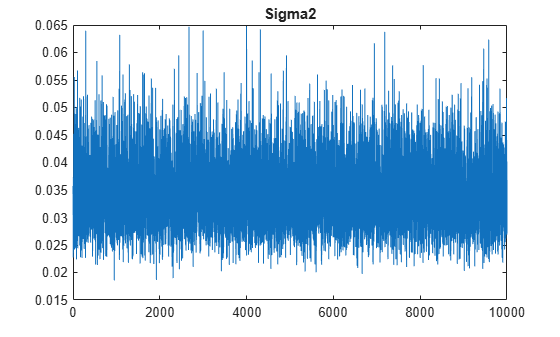The trace plots indicate that the draws seem to mix well. The plot shows no detectable transience or serial correlation, and the draws do not jump between states.

Plot the posterior distributions of the coefficients and disturbance variance.

```figure; plot(PosteriorMdl)```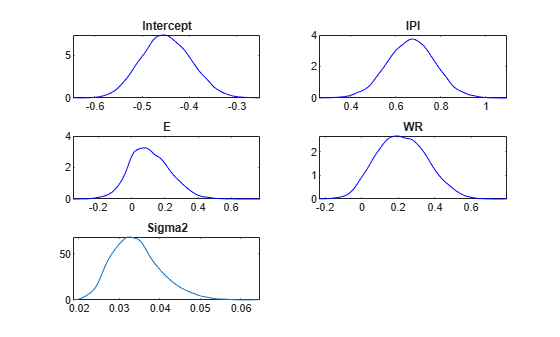`E` and `WR` might not be important predictors because 0 is within the region of high density in their posterior distributions.

Consider the linear regression model in Create Prior Model for Bayesian Lasso Regression and its implementation in Perform Variable Selection Using Default Lasso Shrinkage.

When you implement lasso regression, a common practice is to standardize variables. However, if you want to preserve the interpretation of the coefficients, but the variables have different scales, then you can perform differential shrinkage by specifying a different shrinkage for each coefficient.

Create a prior model for performing Bayesian lasso regression. Specify the number of predictors `p` and the names of the regression coefficients.

```p = 3; PriorMdl = bayeslm(p,'ModelType','lasso','VarNames',["IPI" "E" "WR"]);```

Load the Nelson-Plosser data set. Create variables for the response and predictor series. Determine whether the variables have exponential trends by plotting each in separate figures.

```load Data_NelsonPlosser X = DataTable{:,PriorMdl.VarNames(2:end)}; y = DataTable{:,'GNPR'}; figure; plot(dates,y) title('GNPR')```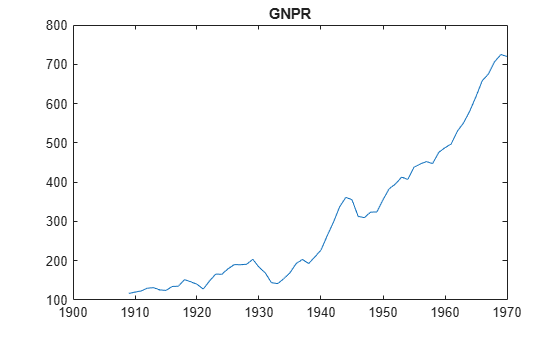```for j = 1:3 figure; plot(dates,X(:,j)); title(PriorMdl.VarNames(j + 1)); end```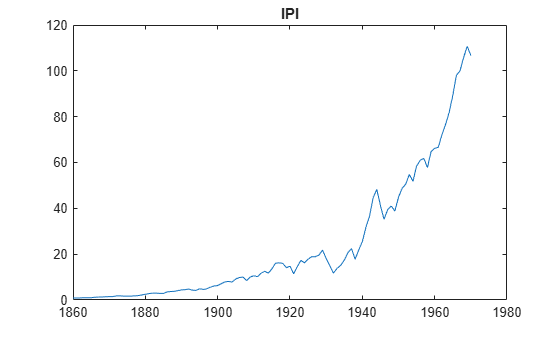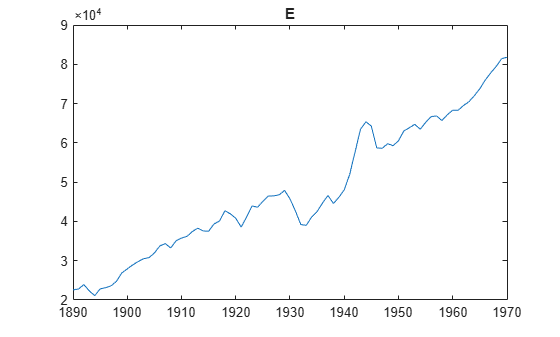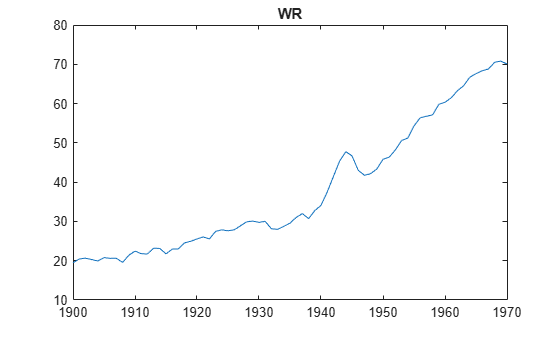The variables `GNPR`, `IPI`, and `WR` appear to have an exponential trend.

Remove the exponential trend from the variables `GNPR`, `IPI`, and `WR`.

```y = log(y); X(:,[1 3]) = log(X(:,[1 3]));```

All predictor variables have different scales (for more details, enter `Description` at the command line). Display the mean of each predictor. Remove observations containing leading missing values from all predictors.

`predmeans = mean(X,'omitnan')`
```predmeans = 1×3 104 × 0.0002 4.7700 0.0004 ```

The values of the second predictor are much greater than those of the other two predictors and the response. Therefore, the regression coefficient of the second predictor can appear close to zero.

Using dot notation, attribute a very low shrinkage to the intercept, a shrinkage of 0.1 to the first and third predictors, and a shrinkage of 1000 to the second predictor.

`PriorMdl.Lambda = [1e-5 0.1 1e4 0.1];`

Implement Bayesian lasso regression by estimating the marginal posterior distributions of $\beta$ and ${\sigma }^{2}$. Because Bayesian lasso regression uses MCMC for estimation, set a random number seed to reproduce the results.

```rng(1); PosteriorMdl = estimate(PriorMdl,X,y);```
```Method: lasso MCMC sampling with 10000 draws Number of observations: 62 Number of predictors: 4 | Mean Std CI95 Positive Distribution ---------------------------------------------------------------------- Intercept | 2.0281 0.6839 [ 0.679, 3.323] 0.999 Empirical IPI | 0.3534 0.2497 [-0.139, 0.839] 0.923 Empirical E | 0.0000 0.0000 [-0.000, 0.000] 0.762 Empirical WR | 0.5250 0.3482 [-0.126, 1.209] 0.937 Empirical Sigma2 | 0.0315 0.0055 [ 0.023, 0.044] 1.000 Empirical ```

Consider the linear regression model in Create Prior Model for Bayesian Lasso Regression.

Perform Bayesian lasso regression:

1. Create a Bayesian lasso prior model for the regression coefficients and disturbance variance. Use the default shrinkage.

2. Hold out the last 10 periods of data from estimation.

3. Estimate the marginal posterior distributions.

```p = 3; PriorMdl = bayeslm(p,'ModelType','lasso','VarNames',["IPI" "E" "WR"]); load Data_NelsonPlosser fhs = 10; % Forecast horizon size X = DataTable{1:(end - fhs),PriorMdl.VarNames(2:end)}; y = DataTable{1:(end - fhs),'GNPR'}; XF = DataTable{(end - fhs + 1):end,PriorMdl.VarNames(2:end)}; % Future predictor data yFT = DataTable{(end - fhs + 1):end,'GNPR'}; % True future responses rng(1); % For reproducibility PosteriorMdl = estimate(PriorMdl,X,y,'Display',false);```

Forecast responses using the posterior predictive distribution and the future predictor data `XF`. Plot the true values of the response and the forecasted values.

```yF = forecast(PosteriorMdl,XF); figure; plot(dates,DataTable.GNPR); hold on plot(dates((end - fhs + 1):end),yF) h = gca; hp = patch([dates(end - fhs + 1) dates(end) dates(end) dates(end - fhs + 1)],... h.YLim([1,1,2,2]),[0.8 0.8 0.8]); uistack(hp,'bottom'); legend('Forecast Horizon','True GNPR','Forecasted GNPR','Location','NW') title('Real Gross National Product: 1909 - 1970'); ylabel('rGNP'); xlabel('Year'); hold off```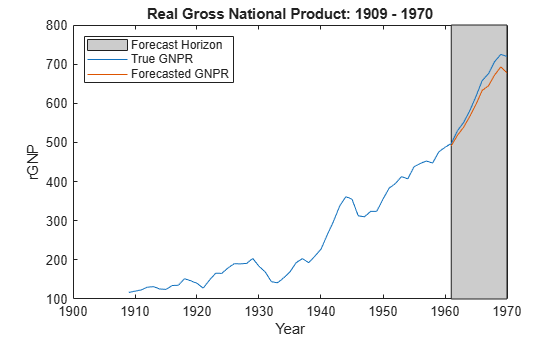`yF` is a 10-by-1 vector of future values of real GNP corresponding to the future predictor data.

Estimate the forecast root mean squared error (RMSE).

`frmse = sqrt(mean((yF - yFT).^2))`
```frmse = 25.4831 ```

The forecast RMSE is a relative measure of forecast accuracy. Specifically, you estimate several models using different assumptions. The model with the lowest forecast RMSE is the best-performing model of the ones being compared.

When you perform Bayesian lasso regression, a best practice is to search for appropriate shrinkage values. One way to do so is to estimate the forecast RMSE over a grid of shrinkage values, and choose the shrinkage that minimizes the forecast RMSE.

expand all

## Tips

• `Lambda` is a tuning parameter. Therefore, perform Bayesian lasso regression using a grid of shrinkage values, and choose the model that best balances a fit criterion and model complexity.

• For estimation, simulation, and forecasting, MATLAB® does not standardize predictor data. If the variables in the predictor data have different scales, then specify a shrinkage parameter for each predictor by supplying a numeric vector for `Lambda`.

## Alternative Functionality

The `bayeslm` function can create any supported prior model object for Bayesian linear regression.

 Park, T., and G. Casella. "The Bayesian Lasso." Journal of the American Statistical Association. Vol. 103, No. 482, 2008, pp. 681–686.